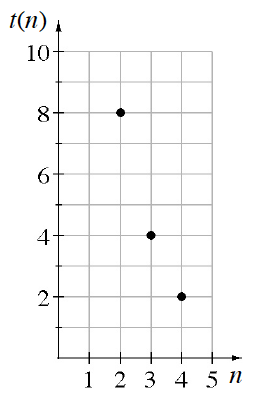### Home > CC3MN > Chapter 6 Unit 10 > Lesson INT1: 6.2.3 > Problem6-77

6-77.

Write an explicit equation for the sequence graphed at right.The multiplier for this graph is $\frac{1}{2}$

Work backward to find term $0$, or the starting value.

Use the starting value and the multiplier to write the equation.

$t(n)=32\left( \frac{1}{2} \right)^n$